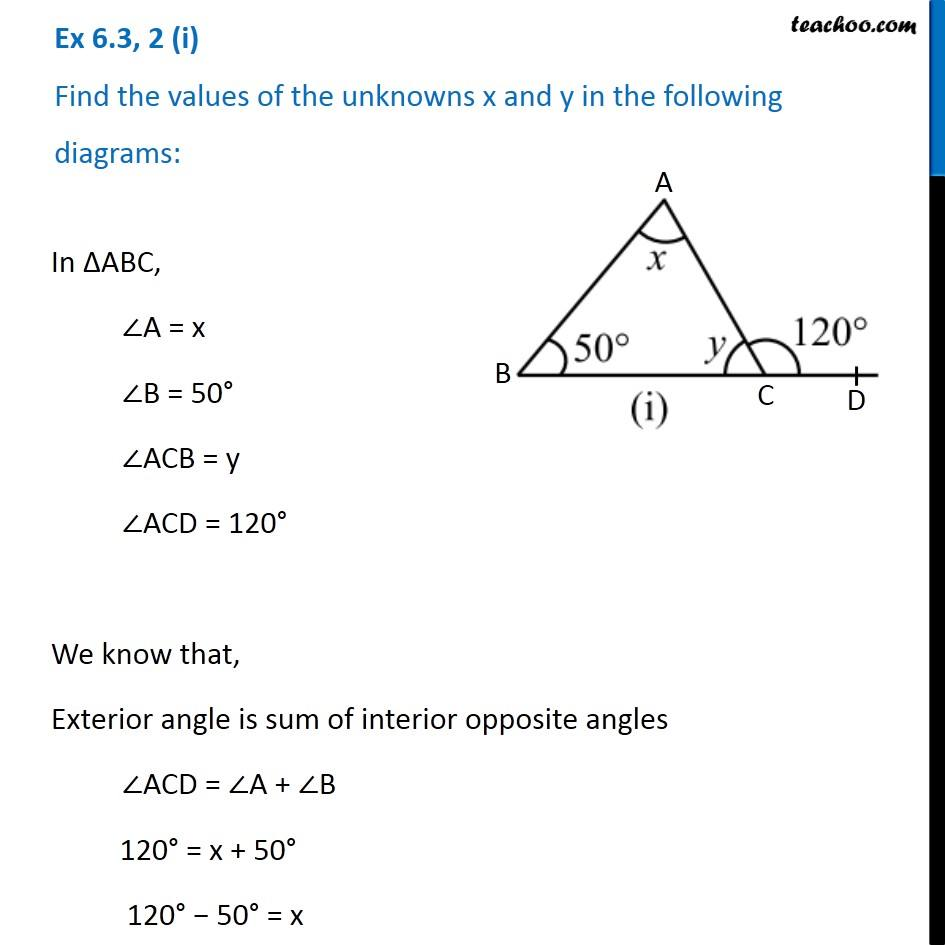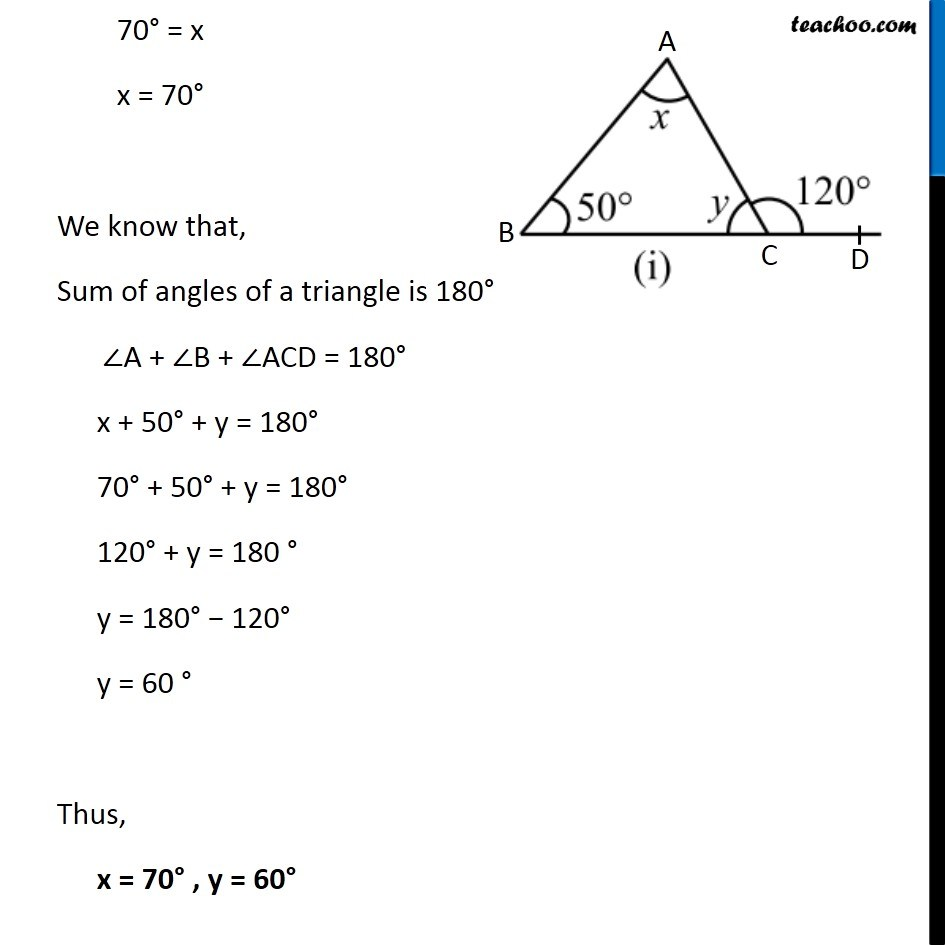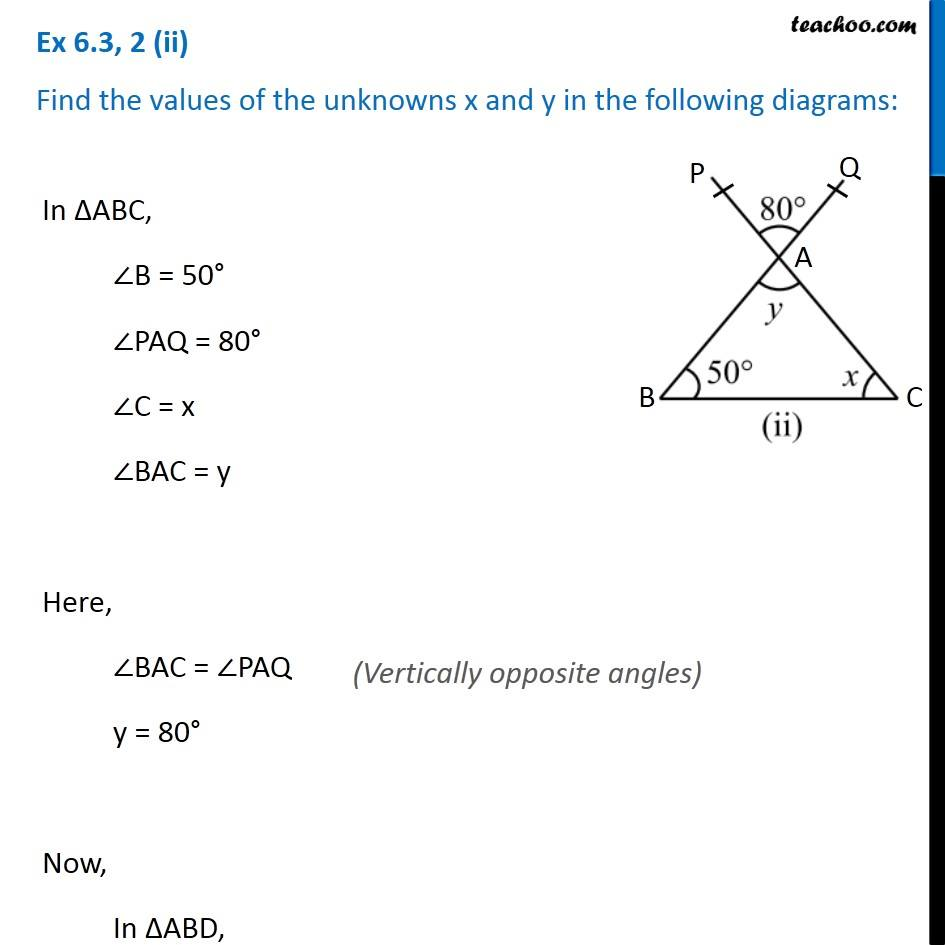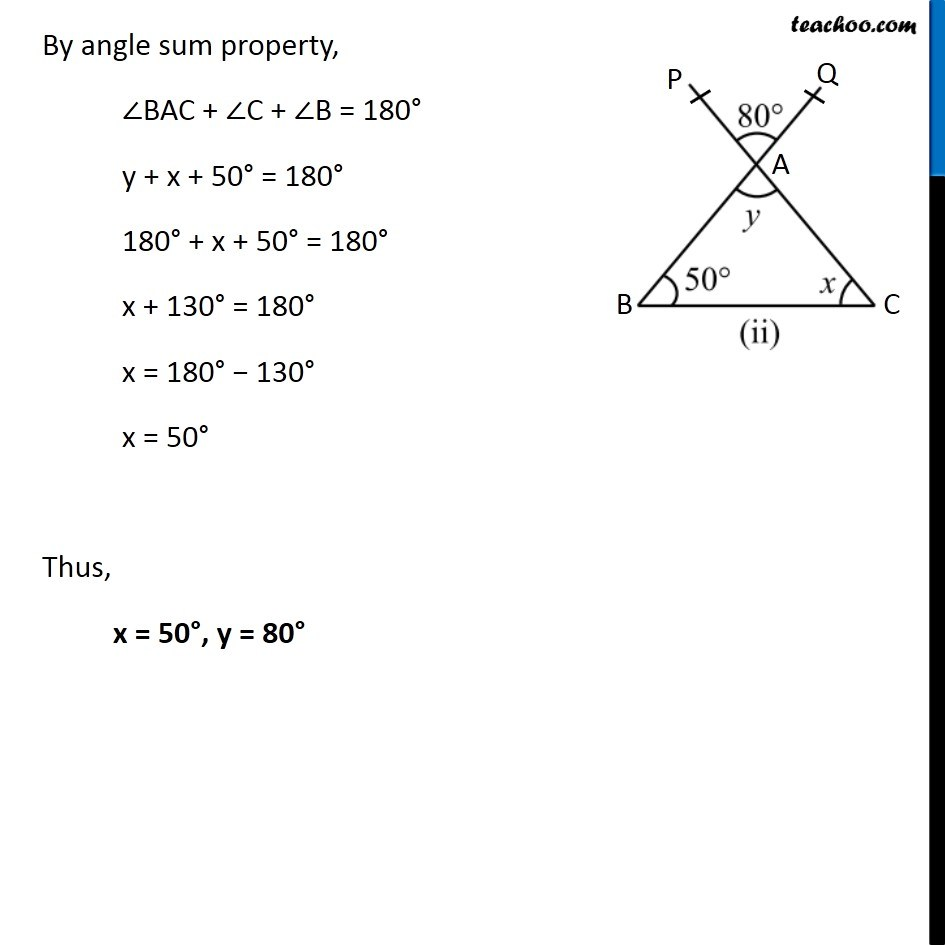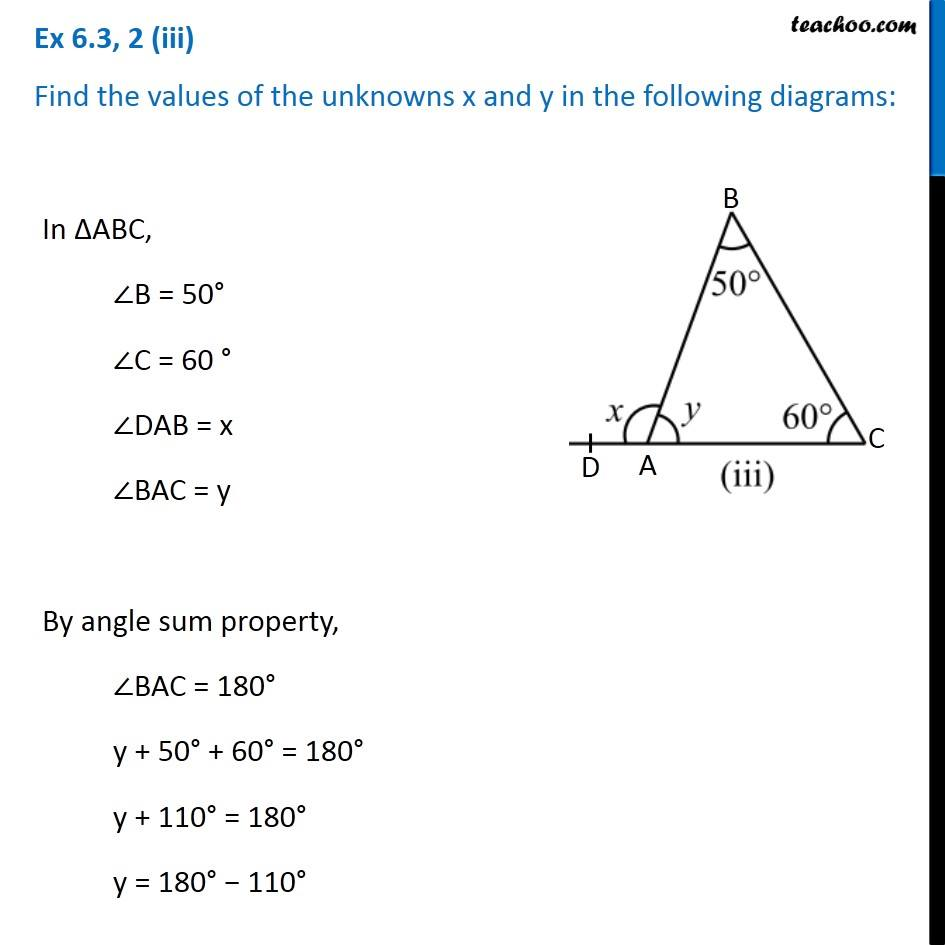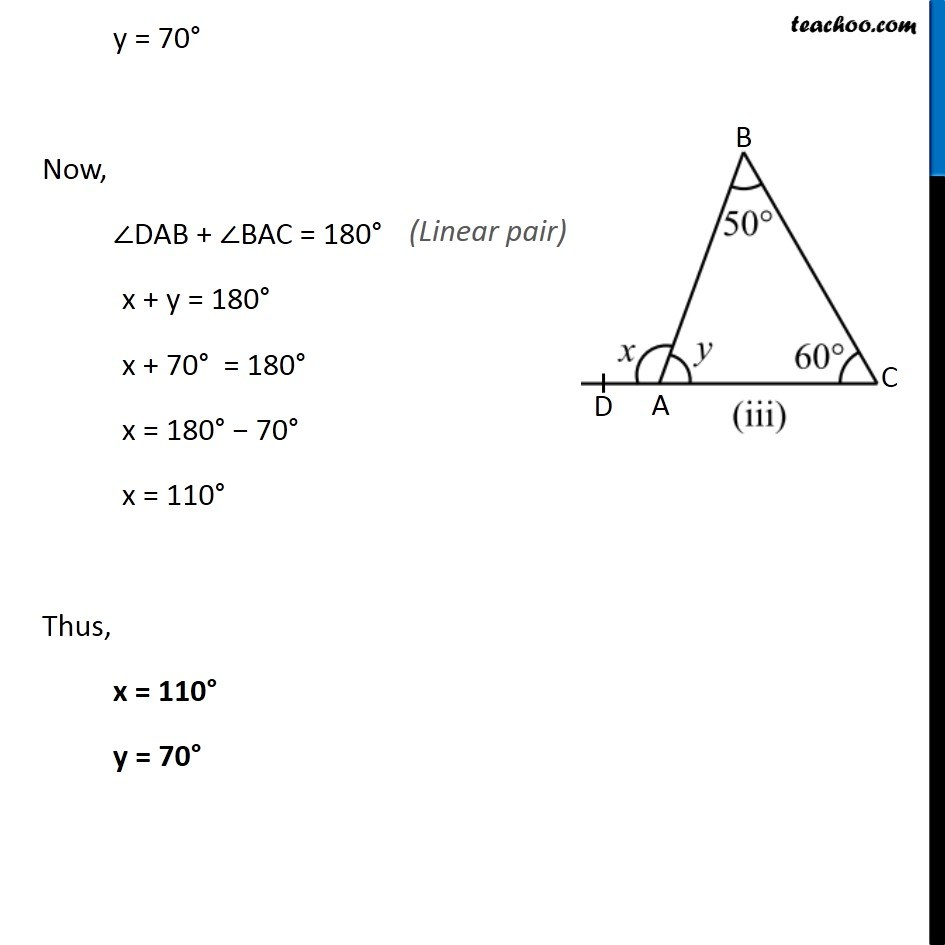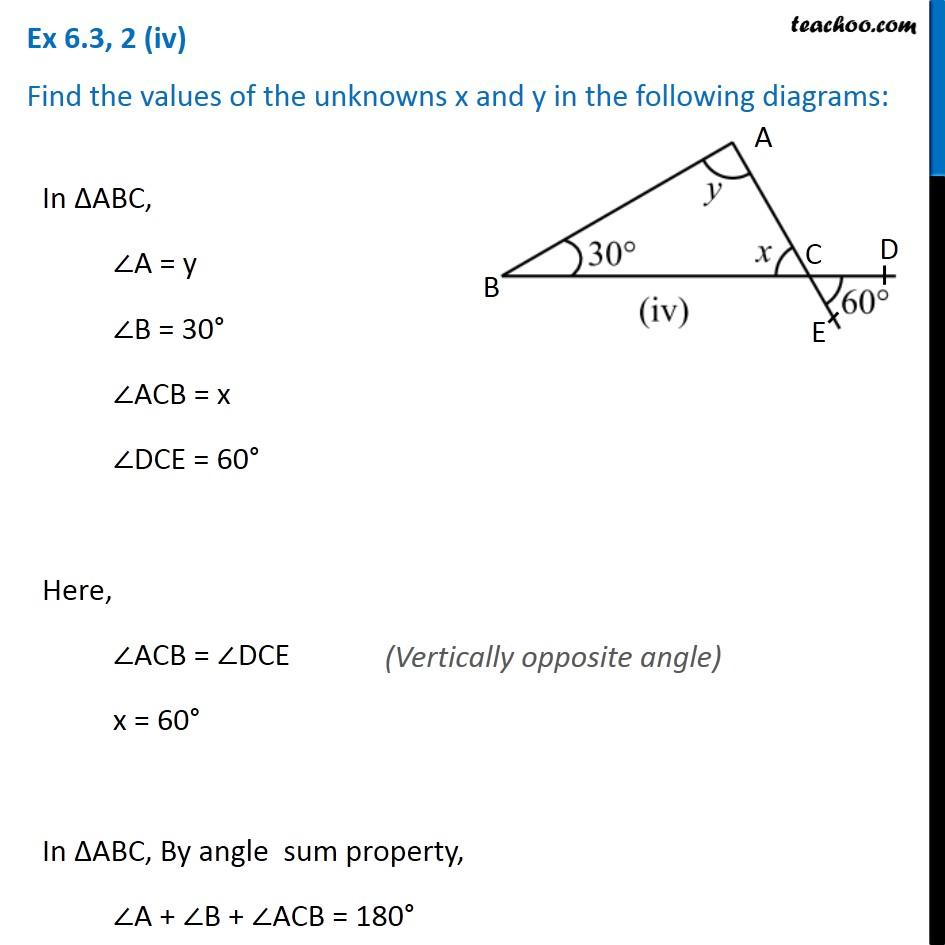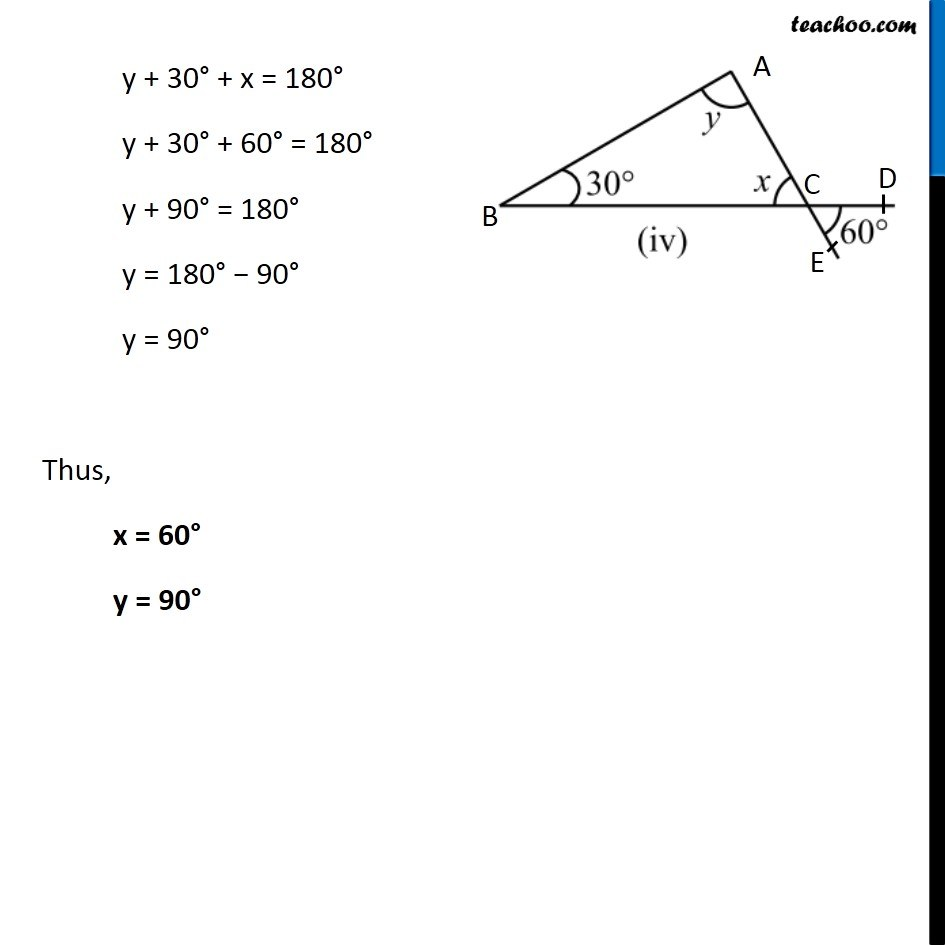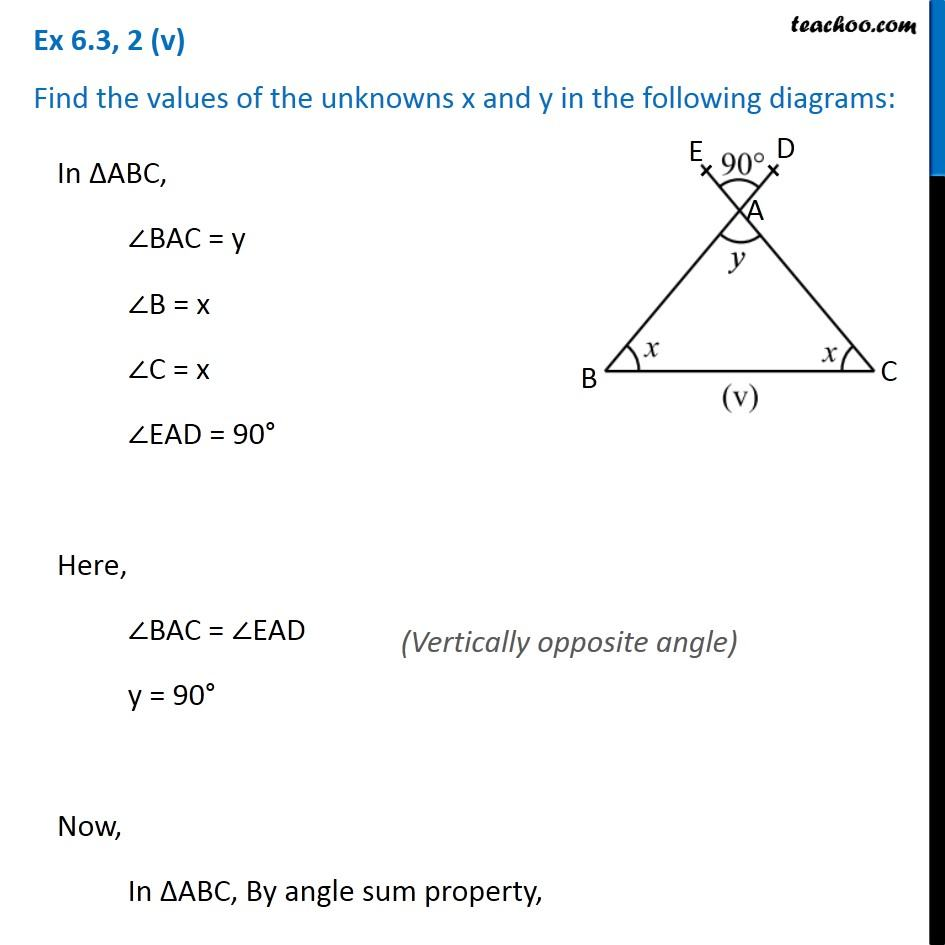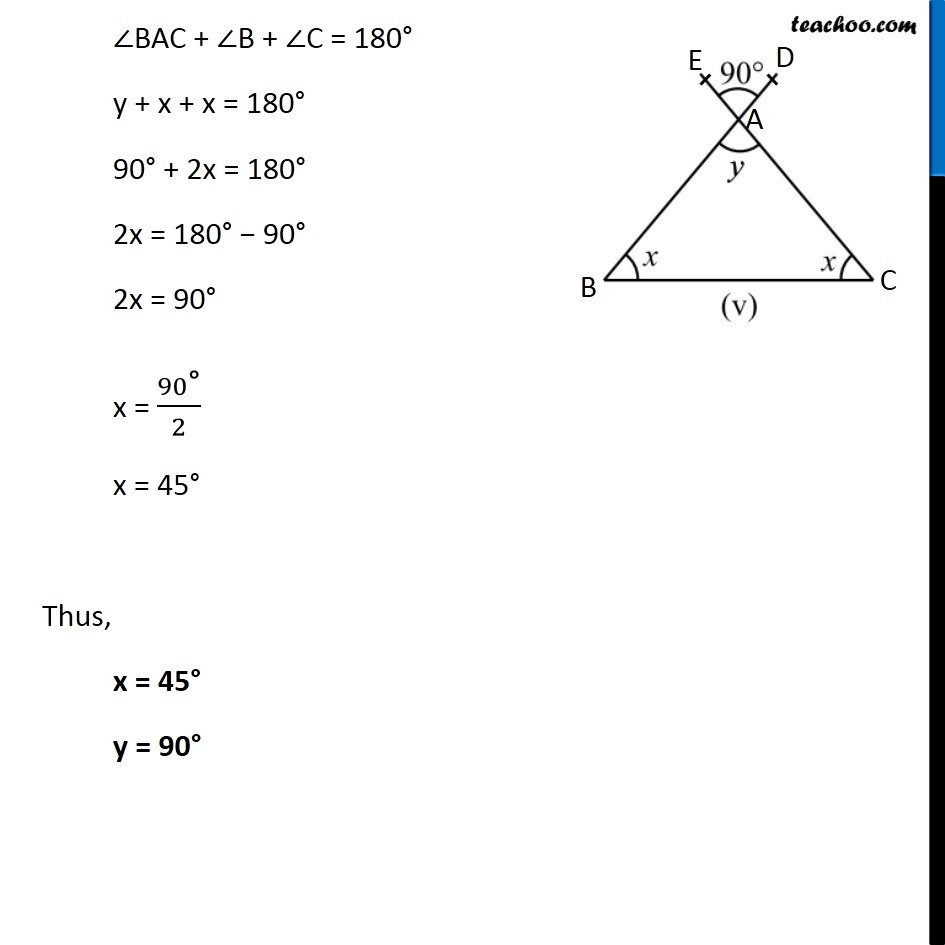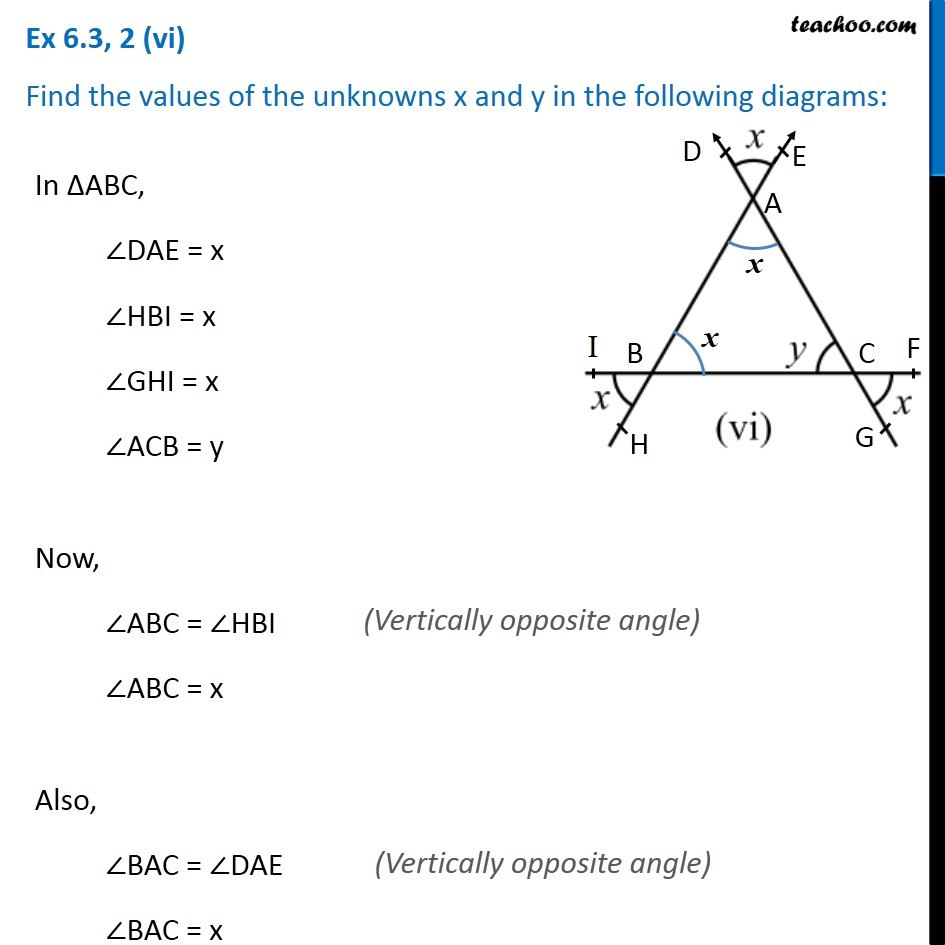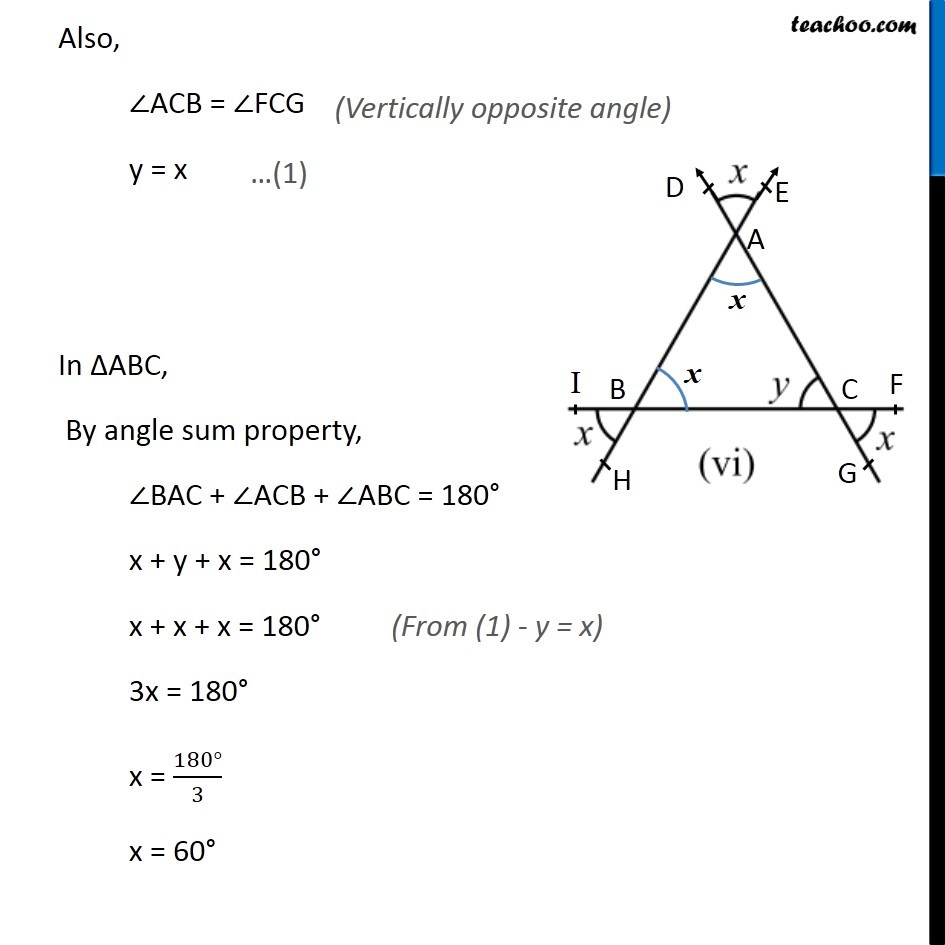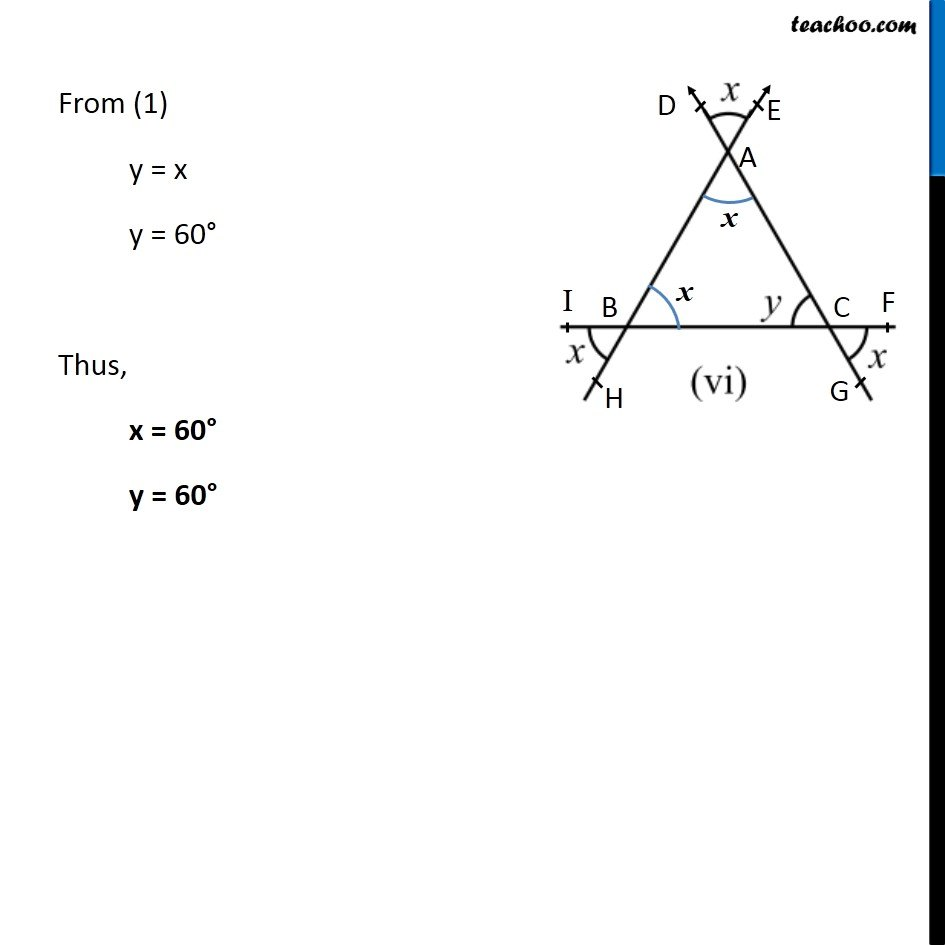Subscribe to our Youtube Channel - https://you.tube/teachoo

1. Chapter 6 Class 7 Triangle and its Properties
2. Serial order wise
3. Ex 6.3

Transcript

Ex 6.3, 2 (i) Find the values of the unknowns x and y in the following diagrams: In ∆ABC, ∠A = x ∠B = 50° ∠ACB = y ∠ACD = 120° We know that, Exterior angle is sum of interior opposite angles ∠ACD = ∠A + ∠B 120° = x + 50° 120° − 50° = x 70° = x x = 70° We know that, Sum of angles of a triangle is 180° ∠A + ∠B + ∠ACD = 180° x + 50° + y = 180° 70° + 50° + y = 180° 120° + y = 180 ° y = 180° − 120° y = 60 ° Thus, x = 70° , y = 60° Ex 6.3, 2 (ii) Find the values of the unknowns x and y in the following diagrams:In ∆ABC, ∠B = 50° ∠PAQ = 80° ∠C = x ∠BAC = y Here, ∠BAC = ∠PAQ y = 80° Now, In ∆ABD, By angle sum property, ∠BAC + ∠C + ∠B = 180° y + x + 50° = 180° 180° + x + 50° = 180° x + 130° = 180° x = 180° − 130° x = 50° Thus, x = 50°, y = 80° Ex 6.3, 2 (iii) Find the values of the unknowns x and y in the following diagrams:In ∆ABC, ∠B = 50° ∠C = 60 ° ∠DAB = x ∠BAC = y By angle sum property, ∠BAC = 180° y + 50° + 60° = 180° y + 110° = 180° y = 180° − 110° y = 70° Now, ∠DAB + ∠BAC = 180° x + y = 180° x + 70° = 180° x = 180° − 70° x = 110° Thus, x = 110° y = 70° Ex 6.3, 2 (iv) Find the values of the unknowns x and y in the following diagrams: In ∆ABC, ∠A = y ∠B = 30° ∠ACB = x ∠DCE = 60° Here, ∠ACB = ∠DCE x = 60° In ∆ABC, By angle sum property, ∠A + ∠B + ∠ACB = 180° y + 30° + x = 180° y + 30° + 60° = 180° y + 90° = 180° y = 180° − 90° y = 90° Thus, x = 60° y = 90° Ex 6.3, 2 (v) Find the values of the unknowns x and y in the following diagrams: In ∆ABC, ∠BAC = y ∠B = x ∠C = x ∠EAD = 90° Here, ∠BAC = ∠EAD y = 90° Now, In ∆ABC, By angle sum property, ∠BAC + ∠B + ∠C = 180° y + x + x = 180° 90° + 2x = 180° 2x = 180° − 90° 2x = 90° x = 90"°" /2 x = 45° Thus, x = 45° y = 90° Ex 6.3, 2 (vi) Find the values of the unknowns x and y in the following diagrams:In ∆ABC, ∠DAE = x ∠HBI = x ∠GHI = x ∠ACB = y Now, ∠ABC = ∠HBI ∠ABC = x Also, ∠BAC = ∠DAE ∠BAC = x Also, ∠ACB = ∠FCG y = x In ∆ABC, By angle sum property, ∠BAC + ∠ACB + ∠ABC = 180° x + y + x = 180° x + x + x = 180° 3x = 180° x = (180°)/3 x = 60° From (1) y = x y = 60° Thus, x = 60° y = 60°

Ex 6.3